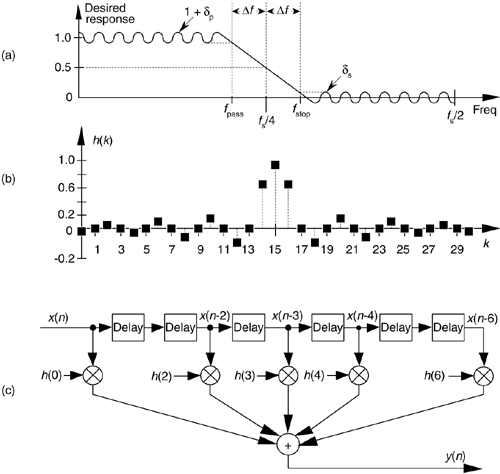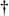# HALF-BAND FIR FILTERS

There's a specialized FIR filter that's proved useful in decimation applications[21–25]. Called a half-band FIR filter, its frequency response is symmetrical about the fs/4 point as shown in Figure 5-33(a). As such, the sum of fpass and fstop is fs/2. This symmetry has the beautiful property that the time-domain FIR impulse response has every other filter coefficient being zero, except at the peak. This enables us to avoid approximately half the number of multiplications when implementing this kind of filter. By way of example, Figure 5-33(b) shows the coefficients for a 31-tap half-band filter where Df was defined to be approximately fs/32 using the Remez Exchange method. (To preserve symmetry, the parameters dp and ds were specified to be equal to each other.)

Figure 5-33. Half-band FIR filter: (a) frequency magnitude response [transition region centered at fs/4]; (b) 31-tap filter coefficients; 7-tap half-band filter structure.Notice how the alternating h(k) coefficients are zero, so we perform 17 multiplications per output sample instead of the expected 31 multiplications. For an S-tap half-band FIR filter, we'll only need perform (S + 1)/2 + 1 multiplications per output sample.[] Be careful though; there's a restriction on the number of coefficients. To build linear phase half-band FIR filters, S + 1 must be an integer multiple of four. The structure of a simple seven-coefficient half-band filter is shown in Figure 5-33(c), with the h(1) and h(5) multipliers absent.

[] Section 13.7 shows a technique to further reduce the number of necessary multiplies for linear phase tapped delay line FIR filters including half-band filters.AmazonPrev don't be afraid of buying books Next